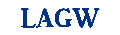# EXCEL ASSIGNMENT HELP

MS Excel Software

Microsoft Excel is a spreadsheet application developed by Microsoft for Microsoft Windows and Mac Operating Systems. It is widely used in making calculations for various works related to mathematics, statistics, finance, and so on. It is a spreadsheet application, where the number of sheets can be added as per our requirements. Each sheet consists of cells with rows and columns, where each cell has different address with respect to row and column.

Many mathematical functions such as sum, product, subtraction and division are available in Excel. Logical functions such as AND, FALSE, IF, IFERROR, NOT, and OR also available in Excel. Similarly, it also includes some financial functions such as ACCRINT, FV, IPMT, IRR, NPV, and so on.

Excel is very much useful for representing the given data in terms of tables and graphs. The main features of Excel includes PivotTable, Table, Picture, Shapes, Clip art, Charts such as bar chart, and scatter plot. Some of the statistical analysis can also be done by the use of Excel and it can be obtained by the 'Data Analysis' tool available under the 'Data' menu. The chart options are available under the 'Insert' menu, by which, one can construct suitable charts such as bar charts, line graphs, and scatter plot with fitted lines.

### Excel Assignment Help

Statisticshelpdesk offers online Excel assignment help to analyse and interpret data. Our Excel Assignment help services comprises of all solution to complex problems associated with Excel software. Our step by step approach helps students to understand the solution themselves. We provide Excel Assignment help through email and chat where a student can simply upload his Excel Homework on our website and get it done before the deadline.

### Excel Homework Help

Excel Homework Help covers all homework and course work questions in Excel. Our tutors are highly efficient in teaching the use and application of Excel software on robust online platform. Students can learn to get the best advantage out of Excel software in solving various statistical problems. Our online Excel Homework help is a one stop solution to get last minute help in exams, practicals, quizzes and tests.

SUBMIT HOMEWORK
• Submit your homework for a free quote
• Name:*
• Email:*
• Phone:*
• Subject:*Change Code